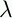September 22, 1999 Perl and the Lambda Calculus Slide #19

# Boolean Value Example

• Goal:

```        (IF TRUE  x y)              => x
```
```        (IF FALSE x y)              => y
```
• Solution:

```        IF :xyz.(x y z)
```
```        TRUE:pq.p
```
```        FALSE:pq.q
```

 NextCopyright © 1999 M-J. Dominus